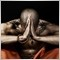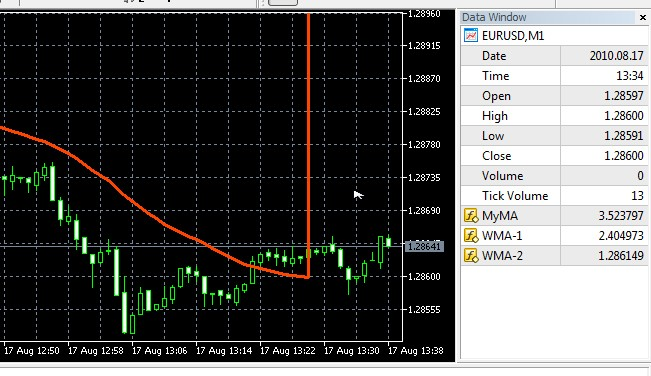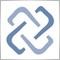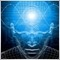# Why does my indicator line flies away?653

Hi Coders!

I'm working on a simple indicator based on two WMA.

When I attach the indicator to the chart, it is drawn well. Don't know why, but after new bars - sometimes at new ticks - my indicator line flies away as you can see the below picture.At the new bars the WMA-1 buffer get false data. In the picture, it's 2.404973. That's why the MyMA get false data too.

The code of the indicator:

```#include <MovingAverages.mqh>

#property indicator_chart_window
#property indicator_buffers 3
#property indicator_plots   3
#property indicator_type1   DRAW_LINE
#property indicator_color1  OrangeRed
#property indicator_width1  3
#property indicator_label1  "MyMA"
#property indicator_label2  "WMA-1"
#property indicator_label3  "WMA-2"

double   HMABuffer[];
double   wma1Buffer[];
double   wma2Buffer[];

input int   Period_1 = 33 ;   // WMA-1 Period
input int   Period_2 = 24 ;   // WMA-2 Period

//+------------------+
//|      OnInit      |
//+------------------+
void OnInit()
{
SetIndexBuffer(0,HMABuffer,INDICATOR_DATA);
SetIndexBuffer(1,wma1Buffer,INDICATOR_DATA);
SetIndexBuffer(2,wma2Buffer,INDICATOR_DATA);
}
//+------------------+
//|   OnCalculate    |
//+------------------+
int OnCalculate(const int rates_total,
const int prev_calculated,
const datetime &Time[],
const double &Open[],
const double &High[],
const double &Low[],
const double &Close[],
const long &TickVolume[],
const long &Volume[],
const int &Spread[]
){

LinearWeightedMAOnBuffer(rates_total,prev_calculated,0,Period_1,Close,wma1Buffer);
LinearWeightedMAOnBuffer(rates_total,prev_calculated,0,Period_2,Close,wma2Buffer);

for ( int i=0; i<rates_total; i++ ){
HMABuffer[i] = 2*wma1Buffer[i]-wma2Buffer[i];
}

return(rates_total);
}```

Where did I take mistake? How can I make it works well?

Thank you.

Relative6654

Most likely you are using old version of MovingAverages.mqh. Arguments count of function LinearWeightedMAOnBuffer have been changed so new version have to show you this error.

Try to download new version of MovingAverages.mqh, fix all errors and warning and try again.653

alexvd:

Most likely you are using old version of MovingAverages.mqh. Arguments count of function LinearWeightedMAOnBuffer have been changed so new version have to show you this error.

Try to download new version of MovingAverages.mqh, fix all errors and warning and try again.

You thought right! My indicator works properly with the new version of MovingAverages.mqh.

Thank you very much alexvd!8

I have the same problem.

For the next ticks, lwma is not calculated. I tried to change for the newest MovingAverages.mql but nothing was change.

An idea ?6654

Morgatte:

I have the same problem.

For the next ticks, lwma is not calculated. I tried to change for the newest MovingAverages.mql but nothing was change.

An idea ?

Are you sure that you are using function LinearWeightedMAOnBuffer correctly? Please look at source code of CHO indicator as an example.8

Hello,

I think I use it correctly (But I am not sure).

So I rewrite it, and my own function works, however it is not still optimized.
There are 2 cycles "For" overlapped, but It is not really a problem because the first make only one cycle between prev_calculated and rates_total in the majority of cases.

When I have try to optimized it, I have had the same problem.

```...
....
LWMA (prev_calculated-1, rates_total-1, (int)myPERIOD, entry_Buffer, exit_Buffer);
....
..
.

//#############################################################################################################################################
int LWMA (int firstIndex, const int lastIndex, const int MMPperiode, const double& entryArray[], double& exitArray[]) {

if (firstIndex < MMPperiode-1) {                                  // Met à 0.0 les membres dont la période est trop grande
for (int i = 0; i < MMPperiode-1; i++) {exitArray[i] = 0.0;}   // pour pouvoir commencer les calcules des moyennes.
firstIndex = MMPperiode-1;                                     //
}                                                                 //

for (int index = firstIndex; index <= lastIndex; index++) {    // Calcule les moyennes mobiles pondérées entre les index [firstIndex, lastIndex] (inclus)
//
double Denominateur = 0;                                    //
double Numerateur = 0;                                      //
int ii = 0;                                                 //
for (int i = index; i > index-MMPperiode; i--) {            //
Denominateur += (MMPperiode-ii);                         //
Numerateur += entryArray[i]*(MMPperiode-ii);             //
ii++;                                                    //
}                                                           //
exitArray[index] = Numerateur/Denominateur;                    //
}                                                              //
return (0);
}
//#############################################################################################################################################

```8

```int LinearWeightedMAOnBuffer(const int rates_total,const int prev_calculated,const int begin,
//const int period,const double& price[],double& buffer[],int &weightsum) {   // <--- Change
const int period,const double& price[],double& buffer[]) {                  // <--- Change
int        i,limit;
double     sum;
int weightsum = period*(period+1)/2;             // <-------------------------- Change

//--- check for data
if(period<=1 || rates_total-begin<period) return(0);
//--- save as_series flags
bool as_series_price=ArrayGetAsSeries(price);
bool as_series_buffer=ArrayGetAsSeries(buffer);
if(as_series_price)  ArraySetAsSeries(price,false);
if(as_series_buffer) ArraySetAsSeries(buffer,false);
//--- first calculation or number of bars was changed
if(prev_calculated==0)
{
//      weightsum=0;                            // <--------------------------- Change
limit=period+begin;
//--- set empty value for first bars
for(i=0;i<limit;i++) buffer[i]=0.0;
//--- calculate first visible value
double firstValue=0;
for(i=begin;i<limit;i++)
{
int k=i-begin+1;
//         weightsum+=k;                         // <------------------------- Change
firstValue+=k*price[i];
}

firstValue/=(double) weightsum;
buffer[limit-1]=firstValue;
}
else {
limit=prev_calculated-1;
}
//--- main loop
for(i=limit;i<rates_total;i++)
{
sum=0;
for(int j=0;j<period;j++) sum+=(period-j)*price[i-j];
buffer[i]=sum/weightsum;
}
//--- restore as_series flags
if(as_series_price)  ArraySetAsSeries(price,true);
if(as_series_buffer) ArraySetAsSeries(buffer,true);
//---
return(rates_total);
}```8

Now that works...3508

Morgatte:

This doesnt work as original lwma function

To add comments, please log in or register